# Algebra 1 : How to multiply monomial quotients

## Example Questions

### Example Question #6 : Simplifying Expressions

Multiply, expressing the product in simplest form: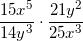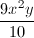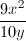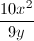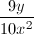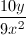Explanation:

Cross-cancel the coefficients by dividing both 15 and 25 by 5, and both 14 and 21 by 7: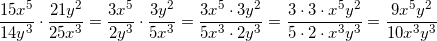Now use the quotient rule on the variables by subtracting exponents: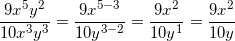### Example Question #7 : Simplifying Expressions

Simplify the following: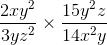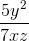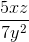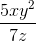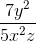Explanation:

In this problem, you have two fractions being multiplied. You can first simplify the coefficients in the numerators and denominators. You can divide and cancel the 2 and 14 each by 2, and the 3 and 15 each by 3: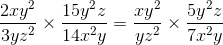You can multiply the two numerators and two denominators, keeping in mind that when multiplying like variables with exponents, you simplify by adding the exponents together: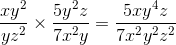Any variables that are both in the numerator and denominator can be simplified by subtracting the numerator's exponent by the denominator's exponent. If you end up with a negative exponent in the numerator, you can move the variable to the denominator to keep the exponent positive: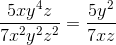### Example Question #11 : Simplifying Exponents

Simplify: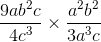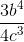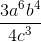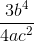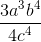Explanation:

In this problem, you have two fractions being multiplied. You can first divide and cancel the coefficients in the numerators and denominators, by dividing 9 and 3 each by 3: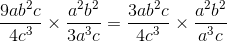Next you can multiply the two numerators, and multiply the two denominators. Remember that when multiplying like variables with exponents, you add the exponents together: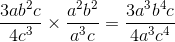If a variable shows up in both the numerator and denominator, you can simplify by subtracting the numerator's exponent by the denominator's exponent. If you end up with a negative exponent in the numerator, you can move the variable and exponent to the denominator to make it positive:### Example Question #13 : Simplifying Exponents

Simplify the following: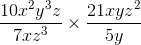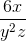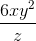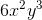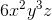Explanation:

In this problem, you have two fractions being multiplied. You can first divide and cancel the coefficients in the numerators and denominators, by dividing 10 and 5 each by 5 and dividing 21 and 7 each by 7: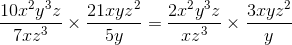Next you can multiply the two numerators, and multiply the two denominators. Remember that when multiplying like variables with exponents, you add the exponents together: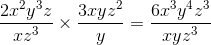If a variable shows up in both the numerator and denominator, you can simplify by subtracting the numerator's exponent by the denominator's exponent: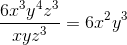### Example Question #15 : Simplifying Exponents

Simplify the following: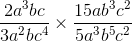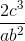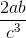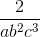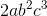Explanation:

In this problem, you have two fractions being multiplied. You can first divide and cancel the coefficients in the numerators and denominators. The two coefficients in the denominators multiply up to 15, allowing you to divide and cancel those two coefficients with the 15 in the numerator: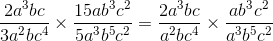Next you can multiply the two numerators, and multiply the two denominators. Remember that when multiplying like variables with exponents, you add the exponents together: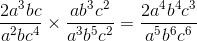If a variable shows up in both the numerator and denominator, you can simplify by subtracting the numerator's exponent by the denominator's exponent. If you end up with a negative exponent in the numerator, you can move the variable and exponent to the denominator to make it positive: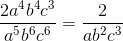### Example Question #32 : Simplifying Expressions

Multiply.  Give the answer in simplest form.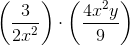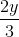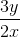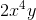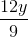Explanation:

Multiplying quotients is similar to multiplying fractions, so we multiply straight across to get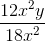. From this point, we can simplify. Since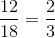and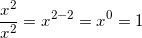, our final answer becomes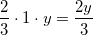.

### Example Question #17 : Simplifying Exponents

Simplify: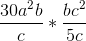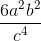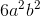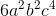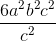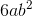Explanation:

Combine like terms in the numerator and the denominator. Use the rules of exponents to combineandin the numerator andandin the denominator. Remember that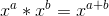Then, divide 30 by 5 (the GCF).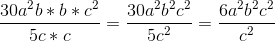The GCF rule can also be used to remove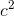from the numerator and the deonominator.goes intoonce.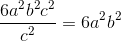### Example Question #41 : Simplifying Expressions

Simplify: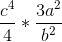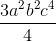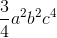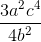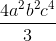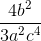Explanation:

Since there are no like terms in the numerator or in the denominator, you can only combine ther terms on the numerator and denominator so that they are in one quotient. You cannot further combine the variables because each variable is represented by a different letter. You cannot further reduce the integers because they do not have a common factor.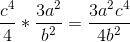### Example Question #4781 : Algebra 1

Multiply the following monomial quotients: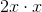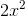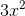Explanation:

To solve this problem, split it into two steps:

1. Multiply the variables. We also need to remember the following laws of exponents rule: When multiplying variables, add the exponents.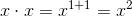2. Combine this with the remaining coefficient to get the final answer: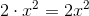### Example Question #4782 : Algebra 1

Multiply the following monomial quotients: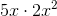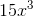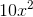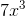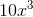Explanation:

To solve this problem, split it into two steps:

1. Multiply the coefficients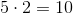2. Multiply the variables. We also need to remember the following laws of exponents rule: When multiplying variables, add the exponents.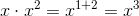Combine these to get the final answer: